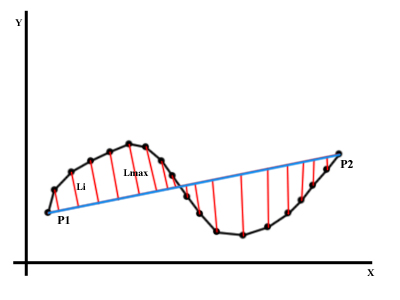## The problem statement

Just imagine the following task. There is a curve consisting of N points in the plane. It is required to construct the curve that follows the contours of the original curve but consists of fewer amounts of points. So, one of the solutions to this problem is to use the approximation algorithms.

Need to perform the approximation of curve A points and obtaining curve B as the result.

Consider the approximation algorithm by the example of a polygon drawn on Google map. As you can see on each polygon boundary there is a large accumulation of points, we can solve this problem by using the algorithm.

After approximation, there are only necessary points on each polygon boundary. Peculiarity of applying the algorithm for map objects is that to achieve a good degree of approximation is necessary to select Emax parameter for each map scale.

## Algorithm description

There is a F function that takes as input a set of K comprising the set of all points of the curve, Emax – the maximum distance from a point to the curve passing through the outermost points of the curve portion. Emax parameter should be chosen empirically, the approximation degree of curve depends on this parameter.

Step by step description of the algorithm:

1. Building a line P1P2 between the extreme points of the set K.

2. Find the most distant point Lmax from the line P1P2.3. If Lmax is more than the specified Emax parameter then divide the set K into two subsets Kl and Kr, relative to the point Lmax and recursively repeat the function F(Kl) and F(Kr) for each of the subsets.

4. If Lmax is less or equal to Emax parameter, then a set R is formed from extreme points of the set K (the start and end points of the curve portion).

## The example of implementation in JavaScript

Below, you can find the implementation of the algorithm in JavaScript language.
``````self.approximate = function (latLngArray, epsilon,
latLngExtractor) {
var approximatedPath = [];
if (latLngArray.length < 3) {
return latLngArray;
}

// Converting coordinates of the start point from the geographic
// system into Cartesian
var startLinePoint = self.convertToCartesian(
latLngExtractor(latLngArray).lat,
latLngExtractor(latLngArray).lng);

// Converting coordinates of the end point from the geographic
// system into Cartesian
var endLinePoint = self.convertToCartesian(
latLngExtractor(latLngArray.slice(-1)).lat,
latLngExtractor(latLngArray.slice(-1)).lng);

// The equation construction of the line, between the start and
// end point in the normal form
var lineEquation = self.buildLineEquation(startLinePoint,
endLinePoint);
var maxDistance = -1;
var maxDistancePointIndex = 0;

// Finding the most distant point from the line
for (var i = 1; i < latLngArray.length - 1; i++) {
var distance =
self.calculateDistanceToLine(self.convertToCartesian(
latLngExtractor(latLngArray[i]).lat,
latLngExtractor(latLngArray[i]).lng), lineEquation);

if (distance != "undefined" && distance >= maxDistance) {
maxDistance = distance;
maxDistancePointIndex = i;
}
}

// If the maximum distance to the line is more than Emax then split
//  the set of coordinates into two subsets and call the function
// recursively
if (maxDistance >= epsilon) {
approximatedPath =
approximatedPath.concat(self.approximate(
latLngArray.slice(0, maxDistancePointIndex + 1),
epsilon, latLngExtractor));

approximatedPath =
approximatedPath.concat(self.approximate(
latLngArray.slice(maxDistancePointIndex),
epsilon, latLngExtractor));

return approximatedPath;
}

// Otherwise return the end points of the curve
else {
return [
latLngArray,
latLngArray[latLngArray.length - 1]
];
}
};

// The equation construction of the line, between the start and
// end point in the normal form
self.buildLineEquation = function (startXY, endXY) {
var aParam = (endXY.y - startXY.y);
var bParam = -1 * (endXY.x - startXY.x);
var cParam = -1 * startXY.x * (endXY.y - startXY.y) +
startXY.y * (endXY.x - startXY.x);
return {a: aParam, b: bParam, c: cParam, startPoint: startXY,
endPoint: endXY};
};

// Finding the distance from a point to a line given by the equation in
// the normal form
self.calculateDistanceToLine = function (pointCoords, lineEquation) {
return Math.abs(lineEquation.a * pointCoords.x +
lineEquation.b * pointCoords.y + lineEquation.c) /
Math.sqrt(Math.pow(lineEquation.a, 2) +
Math.pow(lineEquation.b, 2));
};``````